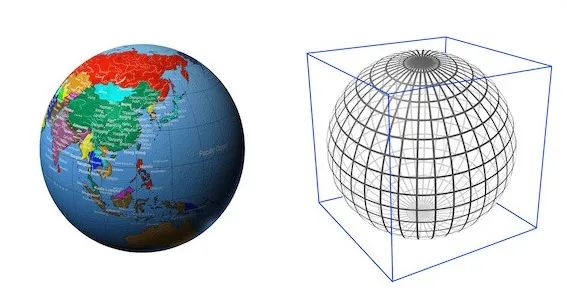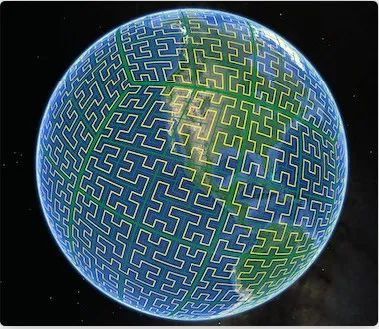features

# Exploring Geospatial Data with NebulaGraph

## What is geospatial data?

Geospatial data is information related to geospatial entities, such as points, lines, and polygons.

NebulaGraph 2.6 supports geospatial data. You can store, compute, and retrieve geospatial data in NebulaGraph. Geography is a data type supported in NebulaGraph. It is composed of latitude and longitude that represents geospatial data.

### How to use geospatial data in NebulaGraph?

Create Schema

The following example shows how to create tags. You can create edge types in the same way.

NebulaGraph currently supports three types of geospatial data: Point, LineString, and Polygon. The following shows how to create geography types and how to insert geospatial data.

``````CREATE TAG any_shape(geo geography);
CREATE TAG only_point(geo geography(point));
CREATE TAG only_linestring(geo geography(linestring));
CREATE TAG only_polygon(geo geography(polygon));
``````

When no geography type is specified, it means that you can store data of any type; when a type is specified, it means that only geospatial data of that type can be stored, such as `geography (point)`, which means that you can only store spatial information of points.

Insert Data

Insert data in the `geo` column of the `any_shape` tag.

``````INSERT VERTEX any_shape(geo) VALUES "101":(ST_GeogFromText("POINT(120.12 30.16)"));
INSERT VERTEX any_shape(geo) VALUES "102":(ST_GeogFromText("LINESTRING(3 8, 4.7 73.23)"));
INSERT VERTEX any_shape(geo) VALUES "103":(ST_GeogFromText("POLYGON((75.3 45.4, 112.5 53.6, 122.7 25.5, 93.9 28.6, 75.3 45.4))"));
``````

Insert data in the `geo` column of the `only_point` tag.

``````INSERT VERTEX only_point(geo) VALUES "201":(ST_Point(120.12，30.16)"));;
``````

Insert data in the `geo` column of the `only_linestring` tag.

``````INSERT VERTEX only_linestring(geo) VALUES "302":(ST_GeogFromText("LINESTRING(3 8, 4.7 73.23)"));
``````

Insert data in the `geo` column of the `only_polygon` tag.

``````INSERT VERTEX only_polygon(geo) VALUES "403":(ST_GeogFromText("POLYGON((75.3 45.4, 112.5 53.6, 122.7 25.5, 93.9 28.6, 75.3 45.4))"));
``````

When the data inserted does not meet the requirements of the specified type, the data insertion fails.

``````(root@nebula) [geo]> INSERT VERTEX only_polygon(geo) VALUES "404":(ST_GeogFromText("POINT((75.3 45.4))"));
[ERROR (-1005)]: Wrong value type: ST_GeogFromText("POINT((75.3 45.4))")
``````

We can see that the geospatial data insertion method is rather peculiar, and is very different from the insertion of basic types such as `int`, `string`, and `bool`.

Let's take `ST_GeogFromText("POINT(120.12 30.16)")` as an example, `ST_GeogFromText` is a geographic location information parsing function, which accepts a string type of geographic location data in WKT (Well-Known Text) standard format.

`POINT(120.12 30.16)` represents a geographic point with longitude 120°12′E and latitude 30°16′N; the `ST_GeogFromText` function parses and constructs a geography data object from the WKT parameter, and then the `INSERT` statement stores it in the NebulaGraph in WKB (Well-Known Binary) standard.

### Geospatial functions

The geospatial functions supported by NebulaGraph can be divided into the following main categories:

• Constructing functions

• `ST_Point(longitude, latitude)`: Constructs a `geography point` object based on a latitude and longitude pair.
• Parsing functions

• `ST_GeogFromText(wkt_string)`: Parses `geography` objects from the WKT text.

• `ST_GeogFromWKB(wkb_string)`: Parses `geography` objects from the WKB text. # Not yet supported, because NebulaGraph does not yet support binary strings.

• Format setting functions

• `ST_AsText(geography)`: Outputs the `geography` object in the WKT text format.

• `ST_AsBinary(geography)`: Outputs the `geography` object in the WKB text format. # Not yet supported, because NebulaGraph does not yet support binary strings.

• Conversion functions

• `ST_Centroid(geography)`: Calculates the center of gravity of a `geography` object, which is a `geography point` object.
• The predicate function

• `ST_Intersects(geography_1, geography_2)`: Determines whether two `geography` objects intersect.

• `ST_Covers(geography_1, geography_2)`: Determines if the first `geography` object completely covers the second.

• `ST_CoveredBy(geography_1, geography_2)`: The inverse of ST_Covers.

• `ST_DWithin(geography_1, geography_2, distance_in_meters)`: Determines if the shortest distance between two `geography` objects is less than the given distance.

• The metric function

• `ST_Distance(geography_1, geography_2)`: Calculates the distance between two `geography` objects.

These function interfaces follow the OpenGIS Simple Feature Access and ISO SQL/MM standards. For details, see NebulaGraph doc.

### Geospatial Index

What is a geospatial index?

Geospatial indexes are indexes that can be used to quickly filter data based on the predicate `ST_Intersects` and `ST_Covers` functions.

NebulaGraph uses the Google S2 library as the geospatial index.

The S2 library projects the Earth's surface into a tangent square, then recursively quadruples each square surface of the square n times, and uses a space-filling curve, the Hilbert curve, to connect the centers of these small square lattices.

When n is infinitely large, this Hilbert curve almost fills the square.

The S2 library uses a Hilbert curve of order 30.The following figure shows that the Earth is filled with Hilbert curves.It can be seen that the Earth's surface is divided into cells by these Hilbert curves. For any geographic shape on the earth's surface, such as a city, a river, or a person's location, we can use several of these cells to completely cover the geographic shape.

Each cell is identified by a unique int64 CellID. Thus, the spatial index of a geographic object is the set of S2 cells that are constructed to completely cover the geographic shape.

When constructing an index of a geospatial object, a collection of different S2 cells that completely cover the indexed object is constructed. The indexing query based on spatial predicate functions quickly filters out a large number of irrelevant geographic objects by finding the intersection between the set of S2 cells that cover the queried object and the S2 cells that cover the indexed object.

Create a geography index

``````CREATE TAG any_shape_geo_index on any_shape(geo)
``````

For geospatial data with the type `point`, it can be represented by an S2 cell of order 30, so a point corresponds to one index entry; for geospatial data with the type `inestring` and `polygon`, we use multiple S2 cells of different levels to cover it, so it will correspond to multiple index entries.

Spatial indexing is used to speed up the lookup of all geo predicates, for example:

``````LOOKUP ON any_shape WHERE ST_Intersects(any_shape.geo, ST_GeogFromText("LINESTRING(3 8, 4.7 73.23)"));
``````

When there is no spatial index on the `geo` column of `any_shape`, this statement will first read all the data of `any_shape` into memory and then use it to calculate whether it intersects with the point (3.0, 8.0), which is generally more expensive. When the amount of data in `any_shape` is large, the computation overhead will be unacceptable.

When the `geo` column of `any_shape` has a spatial index, the statement will first use the spatial index to filter out most of the data that intersected by the line, but there will still be some that may be intersected when read into memory, so there is still one more calculation to be done. In this way, the spatial index quickly filters out most of the data that is not likely to intersect at a small cost, and a small percentage is filtered, greatly reducing the computational overhead.